# Texas Go Math Grade 4 Lesson 18.2 Answer Key Find Profit

Refer to our Texas Go Math Grade 4 Answer Key Pdf to score good marks in the exams. Test yourself by practicing the problems from Texas Go Math Grade 4 Lesson 18.2 Answer Key Find Profit.

## Texas Go Math Grade 4 Lesson 18.2 Answer Key Find Profit

Essential Question

How can you determine if you make a profit?
Answer:  The amount gained by selling a product with more than its cost price. Before you can dive into determining profit margin, you need to know what it is. Your business’s profit margin measures what percentage of revenue your business keeps after paying for outgoing expenses. You can calculate profit margin to see profitability for a specific time period.

To determine your company’s overall profit margin, you’ll want to use the net profit margin formula. Use the formula below to calculate your business’s overall profit margin:

Profit Margin = (Net Income / Revenue) X 100

2. Divide your net income by your revenue (also called net sales)
3. Multiply your total by 100 to get your profit margin percentage.

Unlock the Problem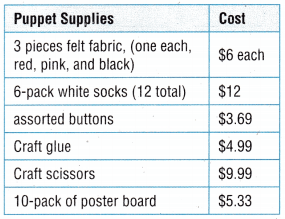Jasmine makes cow sock puppets to earn money to donate to the Future Farmers Club. She buys all of the items shown in the table. Each sock makes one puppet. She will sell the cow puppets for $8 each. Will Jasmine make a profit if she sells all of her puppets? Profit is the amount of money left after all the expenses are subtracted from the amount of money received from selling an item or service. Answer: The cost of cow puppets each=$8
Puppet supplies are already given on the table.
There are 3 pieces of felt fabric $6 each=6*3=$18.
Now add all the supplies=18+12+3.69+4.99+9.99+5.33=$54. If she sells all the cow puppets for$8 each=12*8=$96. {already she has 12 puppets which have to sell for$8 each that’s why I multiplied 8 with 12}.
To know the profit how much she gains, you need to subtract expenses from income. By math sentence, we can write as 96-54=$42. She gained a$42 profit.

Example

(A) Find the total expenses.

Think: Find the cost of the 3 pieces of felt.
Felt: _________ pieces × $___________ =$ ___________.
Add the cost of all the puppet supplies she bought.
_________________________ = ____________
Jasmine spent a total of $_________ on her supplies. Answer: Felt fabric=3 pieces×$6=$18 Now add all the supplies=18+12+3.69+4.99+9.99+5.33=$54.
Jasmine spent a total of $54 on her supplies. (B) Amount received from selling puppets. Jasmine has __________ socks, so she can make __________ puppets. She sells the puppets for$8 each.
Think: to find the amount of money she receives, you must multiply.
__________ puppets × $__________ per puppet =$ __________ .
Jasmine receives __________ from selling all the puppets.
Jasmine has 12 socks. so she can make 12 puppets. She sells each puppet for $8. Now we have to find the amount of money she receives, so you multiply 12 puppets ×$8 per puppet=$96. Jasmine receives$96 from selling all the puppets.

(C) Find the Profit.

Subtract the total expenses from the amount received.
$____96_____ –$ ____54_____ = $___42______ So, Jasmine makes a ___42______ of$ ____96_____ .
To know the profit how much she gains, you need to subtract expenses from income. By math sentence, we can write as 96-54=$42. She gained a$42 profit.

Mathematical Processes
What is the least number of puppets Jasmine has to sell and still make a profit? Explain.
Answer: If she sells 8 puppets for $8 each then 8*8=64$
To know the income we need to subtract income – expenses.
Total expenses=$54 Therefore, 64-54=$10
If she sells 8 puppets then she will gain $10 profit. Share and Show Question 1. Diego runs his own lawn mowing business. His expenses for one week are listed in the chart. He charges his customers$45 to mow a lawn. How much profit did Diego make in the week of June 4th?A. What were his expenses for the week of June 4th?
_________ + _________ + _________ = _________

B. In the week of June 4th, he had 15 customers. How much
money did he take in?
_________ lawns × _________ per lawn = _________

C. What was Diego’s profit? .
Think: Amount received – expenses = profit
_________ – _________ = _________
A. His expenses on June 4th=$67.40+$89+$198.99=$355.39
B. In the week of June 4th, he had 15 customers, by math sentence
15 lawns×$45 per lawn=$675
C. Diego’s profit=675-355.39=$319.69 Question 2. During the week of August 6th, Diego had to buy a new lawn mower for$378. His gas expenses were $45, and his other truck expenses were$89. How much profit did Diego make if he had 12 customers that week?
Expenses: _________ + _________ + _________ = _________
Profit: _________ – _________ = _________
So, Diego made a profit of _________ .
Explanation:
Diego buy a new lawn mower at the cost of $378. The expenses of gas=$45
The expenses of truck=$89 Now add all the expenses. Expense:$378+$45+$89=$512. The question asked was about the profit he gets if he had 12 customers that week. The amount he received on that week=12×$378=$4536. Profit:$4536-$512=$4024. (profit=amount received-expenses)
So, Diego made a profit of $4024. Find the profit. Question 3. Expenses:$222
Earnings: $791 Answer: Formula for profit=Earnings-Expenses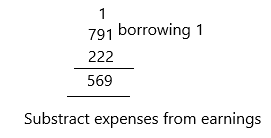Profit=$791-$222 Profit=$569.

Question 4.
Expenses: $96 Earnings:$149.59Formula for profit=Earnings-Expenses
Profit=$149.59-$96
Profit=$53.59. Question 5. Expenses:$195.75
Earnings: $500 Answer: Formula for profit=Earnings-Expenses Profit=$500-$195.75 Profit=$304.25

Question 6.
Expenses: $950.01 Earnings:$1,203.12
Formula for profit=Earnings-Expenses
Profit=$1203.12-$950.01
Profit=$253.11. Question 7. Expenses:$109.90
Earnings: 860
Formula for profit=Earnings-Expenses
Profit=$860-$109.90
Profit=$750.1. Question 8. Expenses:$810.50
Earnings: $2,002.25 Answer: Formula for profit=Earnings-Expenses Profit=$2002.25-$810.50 Profit=$1191.75

Problem Solving

Use the table for 9-11.Question 9.
Multi-Step Ava starts a business making oil paintings of people’s pets. She buys the items shown on the table. She has her paints and other supplies. She charges $75 for an unframed painting. If she sells 20 paintings, how much profit will she make? Answer: The supplies she buys the items are canvas and a master brush set. The cost of canvas=$44.89
The cost of a brush set=$231.21 Now add all the expenses. Expenses=$44.89+$231.21=$276.1
The amount she charged for an unframed painting=$75 The profit she makes, when she sells 20 paintings=20*$75=$1500 Earnings:$1500
Profit=earnings-expenses
profit=$1500-$276.1
profit=$1223.9 Question 10.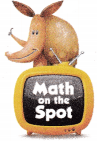H.O.T. What is the least number of paintings Ava needs to sell in order to make a profit? Explain how you found your answer. Answer: 4 paintings. Explanation: I checked with the least numbers which mean in the place of 20, I placed 2, 3 I got no profit and after I placed 4 I got minimum profit. So 4 is the least number of paintings. Ava needs to sell at least 4 paintings to get a profit. Each painting is$75. So I multiplied 75 with 4. By math sentence, I can write as 4*$75=300. I got earnings. I already knew the expenses that are$276.1(check in the above answer).
Profit=earnings-expenses
Profit=300-276.1
Profit=$23.9 Question 11. Apply Ava buys another 20 canvasses. She needs paint, now, so she buys 3 tubes at$11 each. She does not need brushes. What is her profit if she sells 5 paintings?
Answer: $297.11 Explanation: The number of canvasses Ava buys=20 The cost of canvass=$44.89 (Given in the 9th question)
The number of tubes she buys=3
The cost of each tube=$11 The total amount she invested on tubes=11*3=$33
The amount she spends on expense=33+44.89=$77.89 Already in the above question given that the cost of painting she sells that is$75. Once check-in the 9th question.
Now if she sells 5 paintings then she could get 5*$75=$375.
We know expenses and earnings. So, we have to calculate profit.
Profit=earnings-expenses
Profit=375-77.89
Profit=$297.11 Her profit, if she sells 5 paintings is$297.11

Question 12.
H.O.T. Reasoning David and Diane decide to decorate tote bags and sell them. The profit will go to the community garden project. They buy 8 tote bags for $5.93 each and 8 fabric paints for$4.02 each. Estimate the amount they should charge for each tote bag so they make a profit of about $40. Explain. Answer:$2.99 for each bag.
Explanation:
The number of tote bags they buy=8
The cost of each bag=$5.93 The total amount they spend on tote bags=8*$5.93=$47.44 The number of fabric paints they buy=8 The cost of each fabric paint=$4.02
The total amount they spend on fabric paints=8*$4.02=$32.16
The total amount they invested on expenses=47.44+32.16=$79.6 Already profit is given that is$40
Now we need to find out the amount they should charge for each tote bag so they make a profit of about $40. Profit=earnings-expenses.$40= earnings-$79.6 send expenses on the left side$40+$79.6=earnings$119.6=earnings.
The amount they should charge for each bag to make a profit $40 =119.6/40=$2.99.

Question 13.
Jamie builds and sells boxes for worm farms. The materials to build each box cost $13. She sells the boxes for$20 each. How much profit will Jamie make if she sells 5 boxes?
(A) $100 (B)$115
(C) $13 (D)$35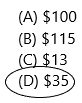Explanation:
The cost of materials to build each box=$13 The amount Jamie invested in materials=13*5=65 The cost of each box Jamie wants to sell=$20
The Jamie earns if she sells 5 boxes=20*5=$100 Now we want to find the profit she gets if she sells 5 boxes. Profit- earnings-expenses Profit=100-65 Profit=$35.

Question 14.
Simon makes a profit of $1.35 on each hot dog he sells. If a hot dog costs him$0.85 to make, how much does Simon sell each hot dog for?
(A) $2.20 (B)$1.35
(C) $0.50 (D)$2.55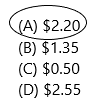Explanation:
The profit Simon makes on each hot dog=$1.35 The cost of each hot dog=$0.85
The earnings she gets after selling each hot dog.
Profit=earnings-expenses
1.35=earnings-0.85
1.35+0.85=earnings
$2.2=earnings. Question 15. Multi-Step Jenny sells her homemade granola bars for$2 each. Each bar costs $1 to make. If she sells 50 granola bars at a street fair, how much profit does Jenny make? (A)$100
(B) $50 (C)$75
(D) $175 Answer: Option B is correct.Explanation: The cost of each homemade granola balls Jenny sells=$2
The amount invested to make each granola ball=$1 The total amount she invested to make=50*=$50
The earnings she could get if she sells 50 granola balls=50*2=$100 Profit=earnings-expenses Profit=100-50 Profit=$50.

TEXAS Test Prep

Question 16.
John has an income tax preparation business. He spent $99 on computer software and$16 on books. He charges each client $25. How much profit can he make if he has 18 clients? (A)$334
(B) $335 (C)$450
(D) $173 Answer: Option B is correct.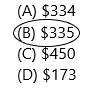Explanation: The amount john invested on the computer=$99
The amount john invested on books=$16 The total amount he invested on xpenses=99+16=$115
The amount he charges for each client=$25 We need to find out the profit if he has 18 clients=25*18=$450
Profit=earnings-expenses
Profit=450-115
Profit=$335. ### Texas Go Math Grade 4 Lesson 18.2 Homework and Practice Answer Key Jackie is making jam to sell at the farmers’ market. Her expenses are shown in the chart.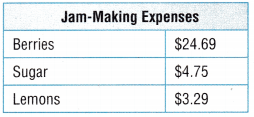Question 1. What are Jackie’s expenses? _______________ + _______________ + _______________ = _______________ Answer:$32.73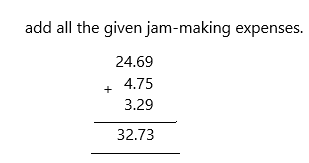Jackie’s expenses are=$24.69+$4.75+$3.29=$32.73

Question 2.
Jackie made 21 jars of jam. She sold all of the jam for $5.50 a jar. How much money did she take in? _______________ × _______________ = _______________ Answer:$115.5
Explanation:
The number of jars Jackie made=21
The cost of the jam of each jar=$5.50 The amount she got after selling all the jars=21*$5.50=$115.5 Question 3. What was Jackie’s profit? _______________ – _______________ = _______________ Answer:$82.77
Explanation:
Formula: Profit=earnings-expenses
Profit=115.5-32.73
Profit=$82.77 Problem Solving Use the table for 4-6.Question 4. Sam and Carla are going to make and sell potholders to earn money for the animal shelter. They need 2 looms, so they will buy 2 loom kits. If they also buy 2 bags of loops and sell the 22 potholders for$4.00 each, how much profit will they make? Explain.
Answer: $12.22 Explanation: The number of loom kits they buy=2 The cost of each loom=$17.94
The total amount they invested on loom kits=2*$17.94=$35.88
The cost of each bag of loops=$19.95 The number of bags they buy=2 The total amount they invested on a bag of loops=2*$19.95=$39.9 The total amount they invested on expenses was$35.88+$39.9=$75.78
If they sell 22 potholders for $4 each, then the profit is 22*$4=$88. Now calculate the profit. Profit=earnings-expenses Profit=$88-$75.78 Profit=$12.22

Question 5.
Sam and Carla decide to buy 2 looms and 10 bags of loops. What is their profit if they sell all 86 potholders for $4.50 each? Answer: Explanation: The number of loom kits they buy=2 The cost of each loom=$17.94
The total amount they invested on loom kits=2*$17.94=$35.88
The cost of each bag of loops=$19.95 The number of bags they buy=10 The total amount they invested on a bag of loops=10*$19.95=$199.5 The total amount they invested on expenses was$35.88+$199.5=$235.38
If they sell 86 potholders for $4.50 each, then the profit is 86*$4.5.0=$387 Now calculate the profit. Profit=earnings-expenses Profit=$387-$235.38 Profit=$151.62

Question 6.
If Sam and Carla buy just the 2 loom kits and sell only the 6 potholders, how much do they need to charge for each potholder to make a profit? Explain.
The number of loom kits they buy=2
The cost of each loom=$17.94 The total amount they invested on loom kits=2*$17.94=$35.88 We can charge from$7 above. I will explain now how it comes. First, look at the expenses they invested and both decided to sell 6 potholders so, once thought if we charge $5 each then there is no profit, there will be a loss. By math sentence, I can write as 6*$5=$30. Profit=$30-$35.88=-5.88 ( They got in minus, there is a loss if they sell$5 each).
For example, charge $8 each then the earnings will be 6*$8=$48. Now calculate profit=earnings-expenses Profit=$48-$35.88 Profit=$12.12
We need to check and calculate the profit and then we have to sell the product.

Lesson Check

Question 7.
Multi-Step Connor sells pretzels for $1.50 each. The pretzels cost$0.45 each to make. How much profit will Connor make if he sells 40 pretzels?
(A) $58.00 (B)$60.00
(C) $78.00 (D)$42.00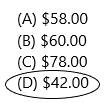The cost of each pretzel to make=$0.45 The total amount Connor invested to make pretzels=40*$0.45=$18 The cost Connor wants to sell each pretzel=$1.50
If he sells 40 pretzels then his earnings are 40*$1.50=$60
Now calculate, profit=earnings-expenses
Profit=$60-$18
Profit=$42. Question 8. Priscilla makes a profit of$24.50 on every scarf she sells. The materials for each scarf cost $8.3. I low much does Priscilla sell each scarf for? (A)$32.89
(B) $16.11 (C)$34.89
(D) $22.21 Answer: Option A is correct.The profit that makes Priscilla on each scarf=$24.50
The cost of materials to make each scarf=$8.3 Now we need to calculate earnings. Profit=earnings-expenses$24.50=earnings-$8.3$24.50+$8.3=earnings$32.8=earnings.
Question 9.
Ivan earned $300.00 doing lawn work. His profit was$266.72. How much were his expenses?
(A) $566.72 (B)$33.28
(C) $144.38 (D)$44.28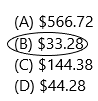The amount Ivan earned was $300.00 The profit Ivan got=$266.72
We need to calculate expenses.
Profit=earnings-expenses
$266.72=$300.00-expenses
$266.72+expenses=$300.00
expenses=300.00-266.72
expenses=$33.28 Question 10. Multi-Step Wilson helped his uncle move. He spent S33.58 for gas and$24.88 for work gloves. He earned $200.00 from his uncle. What is Wilson’s profit? (A)$258.46
(B) $58.46 (C)$141.54
(D) $152.64 Answer: Option C is correct.The total expenses he spent on gas & work gloves=$33.58+$24.88=$58.46
The amount Wilson earned was $200 Calculate, profit=earnings-expenses Profit=200-58.46 Profit=$141.54

Question 11.
Multi-Step Christina sold 35 pairs of gloves for $840. Each pair cost her$6.22 to make. How much profit did Christina make?
(A) $217.70 (B)$622.30
(C) $818.30 (D)$17.78The amount she got after selling 35 pairs of gloves=$840 The cost to make each glove=$6.22
The total amount she invested in making gloves=35*$6.22=$217.7
Profit=$840-$217.7=$622.3 Question 12. Multi-Step Amy sells muffins for$1.35 each. The muffins cost S0.39 each to make. Flow much profit will Amy make if she sells 48 muffins?
(A) $46.08 (B) S69.60 (C)$18.72
(D) $64.80 Answer: Option A is correct.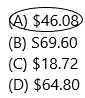The cost of each muffin=$1.35
The cost of each muffin to make=$0.39 The total amount she invested in making muffins=48*$0.39=$18.72 The earnings she gets if she sells 48 muffins=48*$1.35=$64.8 Calculate, profit=earnings-expenses Profit=$64.8-$18.72 Profit=$46.08